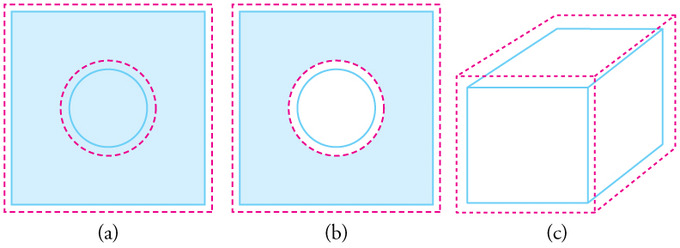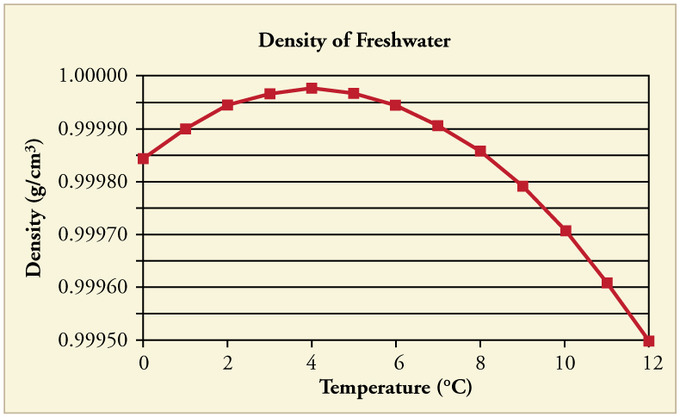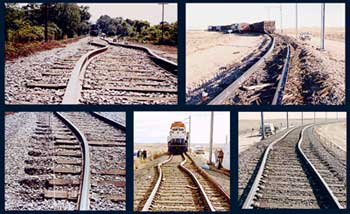## Volume Expansion

Substances expand or contract when their temperature changes, with expansion or contraction occurring in all directions.

The volumetric thermal expansion coefficient is the most basic thermal expansion coefficient. illustrates that, in general, substances expand or contract when their temperature changes, with expansion or contraction occurring in all directions. Such substances that expand in all directions are called isotropic. For isotropic materials, the area and linear coefficients may be calculated from the volumetric coefficient (discussed below).Volumetric Expansion: In general, objects expand in all directions as temperature increases. In these drawings, the original boundaries of the objects are shown with solid lines, and the expanded boundaries with dashed lines. (a) Area increases because both length and width increase. The area of a circular plug also increases. (b) If the plug is removed, the hole it leaves becomes larger with increasing temperature, just as if the expanding plug were still in place. (c) Volume also increases, because all three dimensions increase.

## Relationship to Linear Thermal Expansion Coefficient

For isotropic material, and for small expansions, the linear thermal expansion coefficient is one third the volumetric coefficient. To derive the relationship, let’s take a cube of steel that has sides of length L. The original volume will be V = L3,and the new volume, after a temperature increase, will be:

V+ΔV=(L+ΔL)3=L3+3L2ΔL+3L(ΔL)2+(ΔL)3≈L3+3L2ΔL=V+3VΔLLV+ΔV=(L+ΔL)3=L3+3L2ΔL+3L(ΔL)2+(ΔL)3≈L3+3L2ΔL=V+3VΔLL.

The approximation holds for a sufficiently small ΔLΔL compared to L. Since:

ΔV / V=3ΔL / L

(and from the definitions of the thermal coefficients), we arrive at:

αV=3αLαV=3αL.

## Ice Versus Water

The solid form of most substances is denser than the liquid phase; thus, a block of most solids will sink in the liquid. However, a block of ice floats in liquid water because ice is less dense. Upon freezing, the density of water decreases by about 9%.

## Density of Water as Temperature Changes

At temperatures greater than 4ºC (40ºF) water expands with increasing temperature (its density decreases). However, it expands with decreasing temperature when it is between +4ºC and 0ºC (40ºF to 32ºF). Water is densest at +4ºC.Water Density vs. Temperature: The density of water as a function of temperature. Note that the thermal expansion is actually very small. The maximum density at +4ºC is only 0.0075% greater than the density at 2ºC, and 0.012% greater than that at 0ºC.

Perhaps the most striking effect of this phenomenon is the freezing of water in a pond. When water near the surface cools down to 4ºC it is denser than the remaining water and thus will sink to the bottom. This “turnover” results in a layer of warmer water near the surface, which is then cooled. Eventually the pond has a uniform temperature of 4ºC. If the temperature in the surface layer drops below 4ºC, the water is less dense than the water below, and thus stays near the top.

As a result, the pond surface can completely freeze over, while the bottom may remain at 4ºC. The ice on top of liquid water provides an insulating layer from winter’s harsh exterior air temperatures. Fish and other aquatic life can survive in 4ºC water beneath ice, due to this unusual characteristic of water. It also produces circulation of water in the pond that is necessary for a healthy ecosystem of the body of water.Temperature in a Lake: Temperature distribution in a lake on warm and cold days in winter

## Area Expansion

Objects expand in all dimensions. That is, their areas and volumes, as well as their lengths, increase with temperature.

We learned about the linear expansion (in one dimension) in the previous Atom. Objects expand in all dimensions, and we can extend the thermal expansion for 1D to two (or three) dimensions. That is, their areas and volumes, as well as their lengths, increase with temperature.

## Linear Expansion

To a first approximation, the change in length measurements of an object (linear dimension as opposed to, for example, volumetric dimension) due to thermal expansion is related to temperature change by a linear expansion coefficient. It is the fractional change in length per degree of temperature change. Assuming negligible effect of pressure, we may write:

αL = 1/L dL/dT

where L is a particular length measurement and dL/dT is the rate of change of that linear dimension per unit change in temperature. From the definition of the expansion coefficient, the change in the linear dimension ΔLΔL over a temperature range ΔTΔT can be estimated to be:

ΔL/L = αLΔT.

This equation works well as long as the linear-expansion coefficient does not change much over the change in temperature. If it does, the equation must be integrated.

## Expansion, Not Contraction

Why does matter usually expand when heated? The answer can be found in the shape of the typical particle-particle potential in matter. Particles in solids and liquids constantly feel the presence of other neighboring particles. This interaction can be represented mathematically as a potential curve. Fig 2 illustrates how this inter-particle potential usually takes an asymmetric form rather than a symmetric form, as a function of particle-particle distance. Note that the potential curve is steeper for shorter distance. In the diagram, (b) shows that as the substance is heated, the equilibrium (or average) particle-particle distance increases. Materials which contract or maintain their shape with increasing temperature are rare. This effect is limited in size, and only occurs within limited temperature ranges.

## Linear Expansion

Thermal expansion is the tendency of matter to change in volume in response to a change in temperature.

Thermal expansion is the tendency of matter to change in volume in response to a change in temperature. (An example of this is the buckling of railroad track, as seen in. ) Atoms and molecules in a solid, for instance, constantly oscillate around its equilibrium point. This kind of excitation is called thermal motion. When a substance is heated, its constituent particles begin moving more, thus maintaining a greater average separation with their neighboring particles. The degree of expansion divided by the change in temperature is called the material’s coefficient of thermal expansion; it generally varies with temperature.Fig 1: Thermal expansion of long continuous sections of rail tracks is the driving force for rail buckling. This phenomenon resulted in 190 train derailments during 1998–2002 in the US alone.

## Thermal Expansion

According to the molecular theory of matter, objects absorbing thermal energy have molecules with increasing kinetic energy. This causes the object to expand. All three states of matter undergo thermal expansion. Solids expand in a linear fashion, in an areal fashion (superficial), and in a volumetric (cubical) fashion. Liquids and gases undergo only volumetric expansion. The equations for these thermal expansions are listed below.

• Δ L, Δ A; Δ V represent the change in length, area, and volume, respectively
• α and β are coefficients of expansion
• L represents length
• Δ T represents change in temperature
• n represents moles of gas
• R represents the ideal gas constant

## Equation

This equation describes a linear thermal expansion, which we’ll examine in the context of a metal bar expanding and increasing its length:

In this equation, delta L is the change in length of the bar, delta T is the change in temperature of the bar, L is the original length before the temperature changed, and alpha is the linear coefficient of thermal expansion. The coefficient is just a number that represents how much the material expands. Metals, for example, tend to expand more than plastics.